# CauerLowPassAnalog

Cauer low pass filter with analog components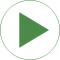# Information

This information is part of the Modelica Standard Library maintained by the Modelica Association.

The example Cauer Filter is a low-pass-filter of the fifth order. It is realized using an analog network. The voltage source V is the input voltage (step), and the R2.p.v is the filter output voltage. The pulse response is calculated.

The simulation end time should be 60. Please plot both V.p.v (input voltage) and R2.p.v (output voltage).

# Parameters (7)

l1 Value: 1.304 Type: Inductance (H) Description: filter coefficient I1 Value: 0.8586 Type: Inductance (H) Description: filter coefficient I2 Value: 1.072 Type: Capacitance (F) Description: filter coefficient c1 Value: 1 / (1.704992 ^ 2 * l1) Type: Capacitance (F) Description: filter coefficient c2 Value: 1.682 Type: Capacitance (F) Description: filter coefficient c3 Value: 1 / (1.179945 ^ 2 * l2) Type: Capacitance (F) Description: filter coefficient c4 Value: 0.7262 Type: Capacitance (F) Description: filter coefficient c5

# Components (11)

G C1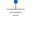Type: Ground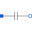Type: CapacitorType: CapacitorType: CapacitorType: CapacitorType: Capacitor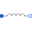Type: InductorType: Inductor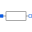Type: ResistorType: Resistor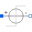Type: StepVoltage